### Numerals

Anomalous numerals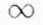((%1000)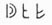((%5000))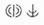((%10000))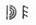((%50000))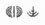((%100000))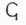((%6))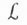((semuncia))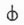((%50-1)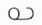((%500000))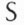((semis))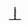((%50-2))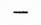((uncia))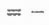((quadrans))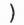((sicilicus))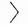((scripulum)) T ((τάλαντον)) M ((μνᾶ)) Stater ((στατήρ)) 𐅂 ((δραχμή)) Obol ((ὀβολός)) 𐅁 ((ἡμιοβόλιον)) 𐅀 o T ((τεταρτημόριον)) X ((χαλκοῦς)) Ounce (gr.) ((οὐγκία)) Λ ((λίτρα)) 𐅂 ((ἡμιλίτριον))